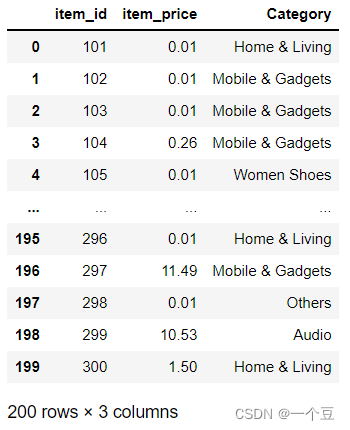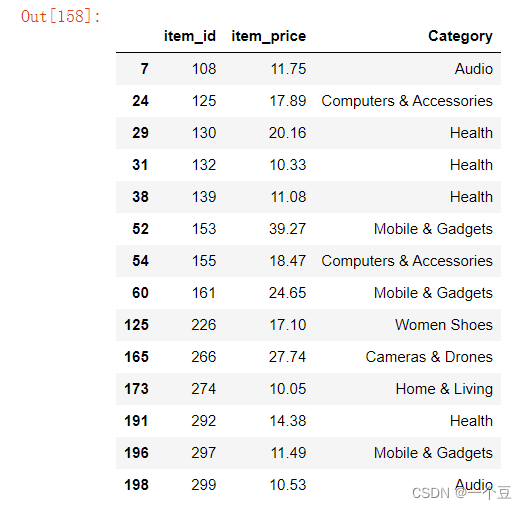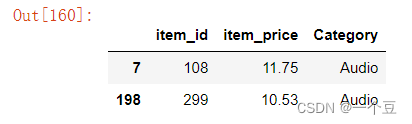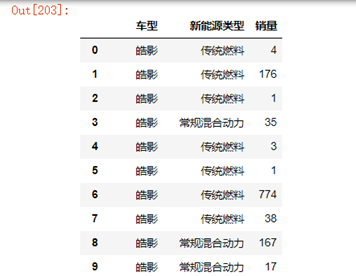• pandas多条件筛选
千次阅读
2021-12-15 19:05:43
• 多条件筛选
gg = g[(g["x偏移"]<1) & (g["x偏移"]>-1)]

更多相关内容
• pandas大于等于&小于等于

有以下item_info表（含字段item_id,item_price及category信息），那么如何输出item_price大于10小于50的全部字段呢？item_info[(item_info['item_price'] >=10) & (item_info['item_price']<50)]e.g.取价格>=10，品类为Audio的数据

item_info[(item_info['item_price'] >=10) & (item_info['Category']=="Audio")]展开全文python
• Pandas多条件筛选数值 正确示范：（注意使用（）和&，不能用and） data[(0.1<data['sentiment']) & (data['sentiment']<0.2)] 错误示范： data[(0.1<data['sentiment']) and (data['sentiment']&...

Pandas多条件筛选数值
正确示范：（注意使用（）和&，不能用and）

data[(0.1<data['sentiment']) & (data['sentiment']<0.2)]


错误示范：

data[(0.1<data['sentiment']) and (data['sentiment']<0.2)]

data[0.1<data['sentiment'] & data['sentiment']<0.2]

data[(0.1<data['sentiment']) and (data['sentiment']<0.2)]

展开全文• 我们在使用pandas操作表格时，通常会使用的筛选功能，但我在网上查找了很资料，pandas貌似没有响应的方法，因此我自己写了一个方法，代码为： import pandas as pd def screen(df,cd): '''表筛选 df：pd....

我们在使用pandas操作表格时，通常会使用到筛选功能，但我在网上查找了很多资料，pandas貌似没有相应的方法，因此我自己写了一个方法，代码为：

import pandas as pd

def df_screen(df,cd):
'''表筛选
df：pd.DataFrame
cd： {字段：[条件1,条件2],} 数据格式字典
'''
df_cy = df.copy()
idx_z = [ True for i in df_cy.index]
orcom  = lambda a,b: [ any([a[i],b[i]]) for i in range(len(a))] # 列表a与列表b两两 或 比较
addcom  = lambda a,b: [ all([a[i],b[i]]) for i in range(len(a))] # 列表a与列表b两两 与 比较
for z in cd:
if isinstance(cd[z],list):
for index,c in enumerate(cd[z]):
if index!=0:
idx_c = orcom(idx_c,list(df_cy[z] == c))
else:
idx_c = list(df_cy[z] == c)

else:
idx_c = list(df_cy[z] == cd[z])
return df_cy.loc[idx_z,:]


数据准备：

test = pd.DataFrame({'车型': ['皓影', '皓影', '皓影', '皓影', '皓影', '皓影', '皓影', '皓影', '皓影', '皓影', '本田CR-V', '本田CR-V', '本田CR-V', '本田CR-V', '本田CR-V', '本田CR-V', '本田CR-V', '本田CR-V'], '新能源类型': ['传统燃料', '传统燃料', '传统燃料', '常规混合动力', '传统燃料', '传统燃料', '传统燃料', '传统燃料', '常规混合动力', '常规混合动力', '传统燃料', '传统燃料', '传统燃料', '传统燃料', '常规混合动力', '常规混合动力', '插电式混合动力', '插电式混合动力'], '销量': [4, 176, 1, 35, 3, 1, 774, 38, 167, 17, 8, 1, 267, 3, 35, 3, 2, 1]})函数调用

cd = {
"车型":["皓影","本田CR-V"], # 需要筛选的字段及筛选的内容
"新能源类型":["传统燃料","常规混合动力"]
}
df_screen(test,cd)


注意：数据为虚构

展开全文python excel 数据挖掘
• 可直接去看 pandas文档 Indexing and selecting data ##df[（条件1）&（条件2）&（条件3）&...]（只能筛选行） df[(df["C"] > 4) & (df["D"] < 4)] ##df.loc[（列条件1）&（列条件2）&...python
• 一是多条件筛选数据，二是将数据写如excel,但是要保存在同一个工作簿的个sheet。 先上一个完整代码 源数据是这样的： 得到的结果是： 我在做的是，得到不同级距（门槛）下‘人数’,‘占比’,‘达到级距...
• 然后删除B列重复的数据，keep参数是只保留第一个，因为是降序排序，第一个B列的C列数据相对于其他重复的C列数据是最大的（有点绕口） 参考： 利用pandas进行条件筛选和组合筛选 - beyondChan - 博客园 Python ...python
• pandas条件复合筛选条件、与、或） 数据分析工作中，Python第三方包pandas功能非常强大，其组合筛选功能比excel速度快很。这里记录一下pandas的组合筛选功能。 或筛选筛选使用的符号是"|" 举个例子： ...python
• ## Python Pandas条件筛选

万次阅读 2022-03-06 14:57:08
import pandas as pd data = pd.read_excel(r'销售数据.xlsx') print(data) 数据如下： 二、以>，<，==，>=，<=来进行选择（“等于”一定是用‘==’，如果用‘=’就不是判断大小了）： 例如：...python 开发语言 后端
• import pandas as pd dt = pd.read_csv(r'C:\Users\wglink\Desktop\out.csv',encoding='gbk').astype(str) citylist = dt['cityname'].drop_duplicates().tolist() for i in citylist: df = dt[(dt['cityname']==i...python
• 1.单条件筛选满足条件的行，然后取某一列 2.多条件筛选 注意小括号不能省略，否则会报错 是否存在某个列表中用isinpython 开发语言 后端
• 在运行代码 print(train_df[(train_df['item_price'] < q1 - 1.5 * iqr) or (train_df['item_price'] > q3 + 1.5 * iqr)]) 时会报错 ValueError: The truth...原因不了解，只需将or改为∣|∣即可，好像进行条件
• import pandas as pd import os os.chdir(r'C:\Users\Hans\Desktop\data_analysis\test_data') df = pd.read_csv('reslut.csv',encoding='gbk') df.head() 日期 收盘 开盘 高 低 百分比变化量 ...
• 下面小编就为大家分享一篇pandas按若干个列的组合条件筛选数据的方法，具有很好的参考价值，希望对大家有所帮助。一起跟随小编过来看看吧
• 主要介绍了pandas条件组合筛选和按范围筛选的示例代码，文中通过示例代码介绍的非常详细，对大家的学习或者工作具有一定的参考学习价值，需要的朋友们下面随着小编来一起学习学习吧
• ## pandas筛选符合多个条件的方法

万次阅读 多人点赞 2019-08-30 19:16:52
时候，我都会面临着筛选出一张表中符合条件的数据，使用pandas可以快速的实现目标，在此记录下自己最常用的筛选手段
• 在SQL语句中进行条件选择使用的是and将条件链接起来，在pandas是可以使用&将条件连接起来。 但是要是注意： 每一个条件要使用括号括起来。 判读是否在一个集合中的时候要用isin。 ...
• 判断条件筛选 1.普通方法筛选 我们首先构造了一个 5X4 的矩阵数据。 import pandas as pd import numpy as np dates = pd.date_range('20200315', periods = 5) df = pd.DataFrame(np.arange(20).reshape(5,4), ......